# What is the ESR of aluminum electrolytic capacitor?

Issuing time:2021-11-04 12:24

ESR parameters of common aluminum electrolytic capacitors

Manufacturers generally have a variety of series of electrolytic capacitors, low ESR, long life, high temperature. And the standard product is the lowest performance, or is the cheapest, the general temperature and life parameters are 85℃/105℃-1000h/2000h. This is the same kind of aluminum electrolytic capacitor I'm talking about here.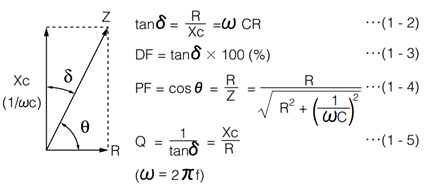The tangent value of loss Angle is the ratio of active power to reactive power. When the frequency is low, such as 120Hz, the inductive reactance can be ignored. Therefore, the formula of loss Angle can be obtained: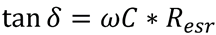The ESR value of ordinary aluminum electrolytic capacitor can not be found in the specifications, but there are loss Angle loss Angle parameters, we can calculate, but this calculation can only be in the case of 120HZ, because in the case of high frequency, ESL can not be ignored, the formula is not applicable.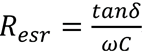However, I found a file of a universal electrolytic capacitor of Nichicon. The ESR of electrolytic capacitor decreases with the increase of frequency, and the rate of change is not large. ESR changes from 120Hz to 100Khz, and the ESR only decreases by half (if you read my previous article on ceramic capacitors, It is found that the ceramic capacitance decreases by about 100-1000 times) as shown below.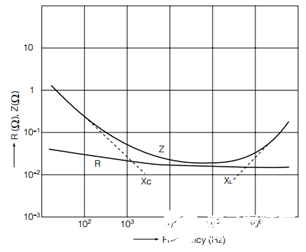The following are the most common aluminum electrolytic capacitor parameters of several brands. The ESR value of 120Hz calculated according to the above method is as follows: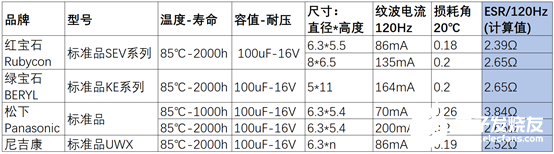We can see that if we limit the capacitance and pressure, the ESR varies little from manufacturer to manufacturer.

In addition, it can also be found that different packaging (size) will not have a great impact on ESR, but will affect the ripple current size, which is easy to figure out, the larger the size, the more heat resistance, the larger the ripple current naturally.

The ESR sizes of aluminum electrolytic capacitors with different capacities and voltages are listed below.

Since each family is similar, take ruby as an example, listed a table for easy inquiry, as shown below.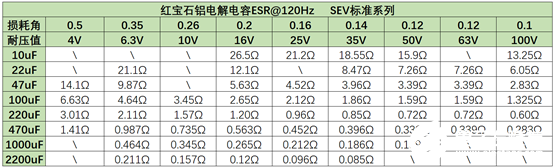It should be noted that this ESR value is at 120Hz. If the frequency increases, ACCORDING to nichicon's file, ESR will decrease somewhat. According to the curve (shown in the previous part of the article), it is about two times (100Khz), but I also found the file provided by Nippon, and ESR decreases more, up to about seven times.

Below is the Nippon curve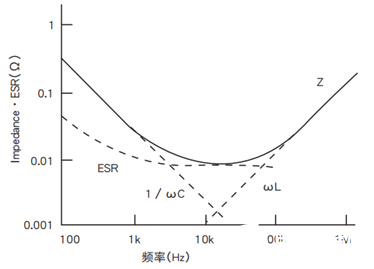Therefore, we can know the ESR of ordinary electrolytic capacitor at 120Hz, but its ESR at 100Khz or other frequencies will be lower, the specific number is not determined.

Low ESR aluminum electrolytic capacitor

In fact, aluminum electrolytic capacitor manufacturers generally provide a number of series of models, applied in different occasions, the above standard series is just one of them, for example, the figure below is panasonic's aluminum electrolytic capacitor series, quite rich, we just need to choose as needed.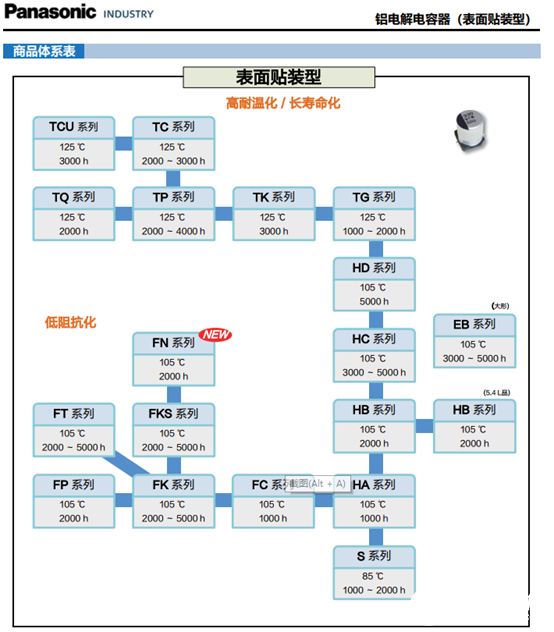Let's look at Low ESR how Low is the ESR of the electrolytic capacitor?

Panasonic FT series 100uF-16V electrolytic capacitor ESR query specification of 0.36 ohms, and table standard series is 2.6 ohms, or much smaller.

Nichikan UCD series 100UF-16V electrolytic capacitor ESR query specification is also 0.36 ohm, Taiwan Lelon corresponding low ESR capacitor is 0.44 ω.

In this way, with the specifications of various manufacturers of low ESR aluminum electrolytic capacitor, ESR value is not much different, are about seven or eight of the standard.

ESR is written here first. In fact, there are many characteristics of aluminum electrolytic capacitors that have not been said, such as the relationship between capacitance and temperature, the relationship between ripple current and temperature, the relationship between ESR and temperature and so on, which are also hardware engineers should know.

In addition, there may be some real-world application problems, such as this one:

In DCDC voltage boost circuit, for example, the output 48V, I want to use electrolytic capacitor filter, because the voltage is too high, the ceramic capacitor capacity can not be too large, the price is very expensive, can electrolytic capacitor standard? After all, Low ESR capacitors are more expensive. Or a combination of a smaller ceramic capacitor and standard electrolytic capacitor? How do you analyze this problem?

Share to：